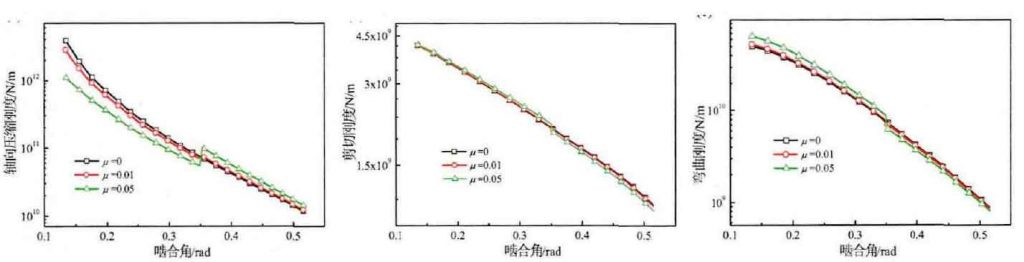# Friction simulation analysis of meshing stiffness of gear transmission system

The basic parameters of gear pair 2 are selected to calculate and analyze the time-varying meshing stiffness with friction effect. Firstly, the influence of friction on the load components along the x-axis and y-axis is studied according to the formula. In order to facilitate data comparison, the load dimension is unified. The curve of load components along the tooth profile is shown in Figure 1.

The dimensionalized load curve in Figure 1 (a) shows that under the action of friction, the load component along the x-axis from the tooth root to the pitch line is slightly less than that without friction, while the load component from the pitch line to the tooth top is slightly greater than that without friction, and with the increase of friction coefficient, the difference between the load component and that without friction is larger, and the difference at different meshing points is not equal. Fig. 1 (b) the load component curve along the y-axis is just opposite to that along the x-axis, that is, the frictional load is greater than the non frictional load from the tooth root to the pitch line, and the frictional load is less than the non frictional load from the pitch line to the tooth top. However, with the increase of friction coefficient, the difference of load components is the same along the c axis (the difference increases and is not equal).(a) Axial compression stiffness (b) Shear stiffness (c) Bending stiffness

According to the load change action formula affected by friction, the compression stiffness Ka, shear stiffness KT and bending stiffness KB shown in the formula are simulated and calculated. The calculation results are shown in Figure 2. Figure 2 (a) shows that the compression stiffness from the root to the pitch line is less than that without friction, while the compression stiffness from the pitch line to the top is greater than that without friction, and the stiffness curve changes obviously with the increase of friction coefficient. The shear stiffness curve in Fig. 2 (b) shows that the compression stiffness from the tooth root to the pitch line is slightly greater than that without friction, while the compression stiffness from the pitch line to the tooth top is slightly less than that without friction. From the visual effect, the effect of friction on the shear stiffness is less than that on the compression stiffness. In Fig. 2 (c), the change trend of bending stiffness curve under friction is similar to that of shear stiffness curve, and the difference between bending stiffness curve and shear stiffness curve increases with the increase of friction coefficient. The above results show that the effect of friction on single stiffness is not the same as increasing or decreasing, but has different effects.